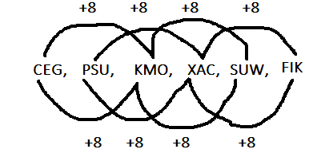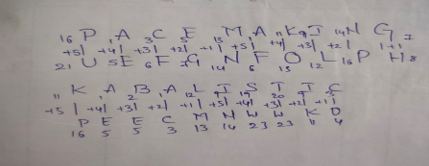# Accenture Abstract Reasoning Previous Year Questions

31 August 2023

Accenture Abstract Reasoning Previous Year Questions

## Get 30 minutes free mentorship by TalentBattle Placement Experts

Cognitive Assessment questions

Technical Assessment

Coding questions

Accenture Abstract Reasoning Previous Year Questions

Q1) Mark the odd one out from the given options.

A. BDG

B. FHK

C. OQT

D. NPT

Explanation:

B+2 -D+3 – G

F+2 – H+3 – K

O+2 – Q+3 – T

N+2 – P+3 – S , the term should be NPS hence option D is the odd man out.

Q2) Mark the option that best completes the comparison.

Sun : Solar System :: Brakes : ?

A. Car

B. Office

C. Recess

D. Time

Explanation: The sun is part of the solar system, Brakes are part of Car.

Q3) Mark the option that best completes the comparison

Rainbow: Sky :: Movie :?

A. Car

B. Picture

C. Theatre

D. Actor

Explanation: Rainbow appear in the sky , Movie is played in Theatre.

Q4) Find the missing term in the series given below:

12, 20, 33, 51, ?, 102

A. 69

B. 82

C. 74

D. 78

Explanation: The series is having an increasing order of +8,+13,+18,+23,+28

51+23 = 74

Q5) Find the missing term in the series given below:

112, 111, 107, 98, ?, 57

A. 83

B. 79

C. 82

D. 87

Explanation: The difference between the numbers are 12 , 22 , 32, 42 and 52

Therefore , 98 – 42 = 82

Q6) Find the missing term in the series given below:

CEG, PSU, KMO, XAC, SUW,?

A. FIK

B. EHJ

C. FHJ

D. JKL

Explanation:Q7) If in a certain code language, “PACEMAKING” is coded as “UEFGNFOLPH”, then how would “KABALISTIC” be coded in the same language?

A. PEECMNWWKD

B. PEEDMNWYKD

C. OEECNMWWKE

D. PEDCNMWWKD

Explanation:Q8) If in a certain code language, “FURNISH” is coded as “GWURNYO”, then how would “MYSTERY” be coded in the same code language?

A.MAUXJXF

B.NAVXHXE

C.NAVXJXF

D.NBFXJXE

Explanation:

The alphabets are in increasing order of +1,+2,+3,+4,+5,+6,-----

Therefore the coded word for MYSTERY is NAVXJXF

Q9) Find the missing term in the series given below. 3, 8, 17, 30, ?, 68

A.47

B.16

C.18

D.25

Explanation:

Difference between the numbers

3           8              17            30              47           68

5           9                  13               17            21

4              4                  4                4

Q10) Find the missing term in the series given below. 5, 6, 13, 26, ?, 70

A.42

B.45

C.41

D.40

Explanation:  Difference between the numbers

6-5= 1

13-6=7

26-13=13

45-26=19

Here, 1,7,13,19 ,25are in AP.

Therefore,

45+25= 70

Q11) If in a certain code language, “URBANISTIC” is coded as “VTEEOKVXJE”, then how “VERMINOSIS” be coded in the same code language?

A.WGHQWEROPI

B.XTYHJUYTDF

C.WGUQJPRWJU

D.WEFGTYULKJ

Explanation:Alphabets are having an increasing order of +1,+2,+3,+4 and again +1,+2,+3,+4
Applying the same logic for VERMINOSIS the coded word will be WGUQJPRWJU

Q12) Find the odd man out in the series : 6, 9, 15, 21, 24, 28, 30

A.28

B.15

C.21

D.30

Explanation: All numbers are multiples of 3 apart of 28.

Q13) Multiple choice (select 1 out of 4 options for the question below)

Identify the missing number in the series 33, ?, 19, 12, 5

A.31

B.26

C.29

D.27

Explanation: From right hand side.

5 + 7 = 12

12 + 7 = 19

19 + 7 = 26

26 + 7 = 33

Q14) Multiple choice ( Select 1 out of 4 options, for the question below.)

Choose the word which is least like the other words in the group:

A.Boy

B.Child

C.Man

Explanation: Child is odd as it is in common gender.

Q15) Multiple choice (select 1 out of 4 options for the questions below)

A girl introduced a boy as the son of the daughter of the father of her uncle. The boy is girl’s?

A.Brother

B.Son

C.Son in Law

D.Nephew

Explanation: Girl's uncle's father = Girl's grandfather (paternal or maternal)

Girl's uncle's father's daughter = Girl's grandfather's daughter = Girl's aunt or girl’s mother.

So, the body is the son of the girl's aunt or girl's mother. The boy and girl could be the cousins or siblings. Thus, the boy would be the brother.

Accenture Pattern and Syllabus

##